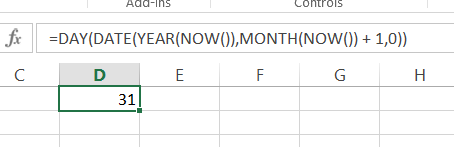### Formula To Get The Number Of Days In The Month

Let’s see how to get the number of days in the month. You may need it to the report or dashboard which you will create in the spreadsheet. There are several ways to create such a formula.

The first formula is:=DAY(DATE(YEAR(NOW()),MONTH(NOW()) + 1,0))The other way I know is:

=DAY(EOMONTH(NOW(),0))Please note that this formula will only for Excel 2007 onwards. For Excel 2003 you can use only the first formula (or move to the newer version of Excel :-)).

```Further reading:
How to calculate monthly payment?
How to Create Rolling 6 Months Average?
How to Format Date and Time?
Turning Off the Automatic Formatting of Dates```

## Template

```Further reading: